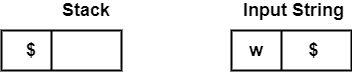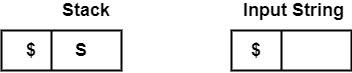# What is Stack Implementation of Shift Reduce Parsing in compiler design?

Shift reduce parser is a type of bottom-up parser. It uses a stack to hold grammar symbols. A parser goes on shifting the input symbols onto the stack until a handle comes on the top of the stack. When a handle occurs on the top of the stack, it implements reduction.

There are the various steps of Shift Reduce Parsing which are as follows −

• It uses a stack and an input buffer.

• Insert $at the bottom of the stack and the right end of the input string in Input Buffer.• Shift: Parser shifts zero or more input symbols onto the stack until the handle is on top of the stack. • Reduce: Parser reduce or replace the handle on top of the stack to the left side of production, i.e., R.H.S. of production is popped, and L.H.S is pushed. • Accept: Step 3 and Step 4 will be repeated until it has identified an error or until the stack includes start symbol (S) and input Buffer is empty, i.e., it contains$.• Error: Signal discovery of a syntax error that has appeared and calls an error recovery routine.

For example, consider the grammar

S → aAcBe
A → Ab|b
B → d

and the string is abbcde.

It can reduce this string to S. It can scan string abbcde looking for the substring that matches the right side of some production. The substrings b and d qualify.

Let us select the left-most b and replace it with A, the left side of the production A → b, and obtain the string aAbcde. It can identify that Ab, b, and d each connect the right side of some production. Suppose this time it can select to restore the substring Ab by A, the left side of the production A → Ab and it can achieve aAcde.

Thus replacing d by B, the left side of the production B → d, and can achieve aAcBe. It can replace this string by S. Each replacement of the right-side of a production by the left side in the process above is known as reduction.

Drawbacks of Shift Reduce Parsing

• Shift|Reduce Conflict − Sometimes, the SR parser cannot determine whether to shift or to reduce.

• Reduce|Reduce conflict − Sometimes, the Parser cannot determine which of Production should be used for reduction.

Example − To stack implementation of shift-reduce parsing is done, consider the grammar −

E → E + E
E → E ∗ E
E → (E)
E → id

and input string as $id_{1} + id_{2} * id_{3}$.

StackInput StringAction
$id1 + id2 * id3$Shift
$id1+id2 * id3$Reduce by E → id
$E+id2 * id3$Shift
$E +id2 * id3$Shift
$E + id2* id3$Reduce by E → id
$E + E* id3$Shift
$E + E *id3$Shift
$E + E * id3$Reduce by E → id
$E + E * E$Reduce by E → E * E
$E + E$Reduce by E → E + E
$E$Accept